Courses

# Projectile MCQ Level - 1

## 40 Questions MCQ Test | Projectile MCQ Level - 1

Description
This mock test of Projectile MCQ Level - 1 for Class 11 helps you for every Class 11 entrance exam. This contains 40 Multiple Choice Questions for Class 11 Projectile MCQ Level - 1 (mcq) to study with solutions a complete question bank. The solved questions answers in this Projectile MCQ Level - 1 quiz give you a good mix of easy questions and tough questions. Class 11 students definitely take this Projectile MCQ Level - 1 exercise for a better result in the exam. You can find other Projectile MCQ Level - 1 extra questions, long questions & short questions for Class 11 on EduRev as well by searching above.
QUESTION: 1

Solution:
QUESTION: 2

Solution:
QUESTION: 3

### A ball is thrown at different angles with the same speedu and from the same point and it has the same rangein both the cases. lf y1 and y2 are the heights attainedin the two cases, then y1+ y2 is equal to

Solution:
QUESTION: 4

The equation of motion of a projectile is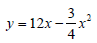The horizontal component of velocity is 3 ms-1. What is the range of the projectile?

Solution:
QUESTION: 5

The range R of projectile is same when its maximumheights are h1 and h2. What is the relation betweenR, h1 ,and h2?

Solution:
QUESTION: 6

The friction of air causes a vertical retardation equalto 10 % of the acceleration due to gravity (take g =10 ms-2). The maximum height will be decreased by

Solution:
QUESTION: 7

The friction of air causes a vertical retardation equal to 10 % of the acceleration due to gravity (take g = 10 ms-2). The maximum height will be decreased by

a. 8 %

b. 9 %

c. 10 %

d. 11 %

Q.

In the above part , the time taken toreach the maximum height will be decreased by

Solution:
QUESTION: 8

The friction of air causes a vertical retardation equal to 10 % of the acceleration due to gravity (take g = 10 ms-2). The maximum height will be decreased by

a. 8 %

b. 9 %

c. 10 %

d. 11 %

Q.

In the above part , the time takento return to the ground from the maximum height

Solution:
QUESTION: 9

At what angle with the horizontal should a ball be thrown so that the range R is related to the time of flight as R = 5T2? (Take g = 10 ms-2)

Solution:
QUESTION: 10

A ball thrown by one player reaches the other in 2 s.The maximum height attained by the ball above the point of projection will be about:

Solution:

Given T is 2 sec

As we know, T = 2uSinθ / g
2 = 2uSinθ/g
uSinθ = g
Squaring on both sides
∴ u²sin²θ = g² — (1)

H = u²Sin²θ/(2g)
From equation (1)
H = g²/(2g)
H = g/2
H = 4.9 m (or) H = 5.0m (if g is taken as 10m/s²)

QUESTION: 11

A ball rolls off the top of a staircase with a horizontal velocity μ ms-1. If the steps are h metre high and b metre wide, the ball will hit the edge of the n th step, if

Solution:
QUESTION: 12

A projectile is projected with initial velocity (6iˆ + 8 ˆj) ms-1. If g = 10 ms-2, then horizontal range is

Solution:
QUESTION: 13

At a height 0.4 m from the ground, the velocity of aprojectile in vector form is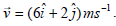The angle of projection is

Solution:
QUESTION: 14

A projectile is thrown in the upward direction makingan angle of 60° with the horizontal direction with avelocity of 150 ms-1. Then the time after which its inclination with the horizontal is 45° is

Solution:
QUESTION: 15

A number of bullets are fired in all possible directionswith the same initial velocity u. The maximum area of ground covered by bullets is

Solution:
QUESTION: 16

A person takes an aim at a monkey sitting on a treeand fires a bullet. Seeing the smoke the monkey beginsto fall freely then the bullet will

Solution:
QUESTION: 17

A person sitting in the rear end of a compartment throwsa ball towards the front end. The ball follows a parabolic path. The train is moving with uniform velocity of 20 ms-1. A person standing outside on the ground also observes the ball. How will the maximum heights (hm)
attained and the ranges (R) seen by the thrower and the outside observer compare each other?

Solution:
QUESTION: 18

Two stones are projected with the same speed butmaking different angles with the horizontal. Their rangesare equal. If the angle of projection of one is p /3 andits maximum height is h1 then the maximum height ofthe other will be

Solution:
QUESTION: 19

A ball is projected from the ground at angle θ with thehorizontal. After 1 s it is moving at angle 45° with thehorizontal and after 2 s it is moving horizontally. Whatis the velocity of projection of the ball?

Solution:
QUESTION: 20

A body is projected horizontally from the top of a towerwith initial velocity 18 ms-1. It hits the ground at angle 45°. What is the vertical component of velocity when strikes the ground?

Solution:
QUESTION: 21

A plane flying horizontally at 100 ms-1 releases an object which reaches the ground in 10 s. At what angle with horizontal it hits the ground?

Solution:
QUESTION: 22

A hose lying on the ground shoots a stream of waterupward at an angle of 60° to the horizontal with thevelocity of 16 ms-1. The height at which the water strikes the wall 8 m away is

Solution:
QUESTION: 23

There are two values of time for which a projectile is atthe same height. The sum of these two times is equalto (T= time of flight of the projectile)

Solution:
QUESTION: 24

A golfer standing on level ground hits a ball with avelocity of u = 52 ms-1 at an angle α above thehorizontal. If tan α = 5/12, then the time for which the ball is at least 15 m above the ground will be (take g = 10 ms-2)

Solution:
QUESTION: 25

The ceiling of a hall is 40 m high. For maximum horizontal distance, the angle at which the ball may be thrown with a speed of 56 ms-1 without hitting the ceiling of the hall is (Take g = 9.8 ms-2)

Solution:
QUESTION: 26

What is the angle of projection for a bullet fired over a horizontal plane, when horizontal range is equal to 4 times the maximum height?

Solution:
QUESTION: 27

A body is projected up along a smooth inclined planewith velocity u from the point A as shown in Fig.The angle of inclination is 45° and the top is connected to a well of diameter 40 m. If the body just manages tocross the well, what is the value of u ? Length of inclinedplane is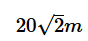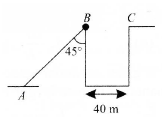Solution:
QUESTION: 28

A rifle shoots a bullet with a muzzle velocity of 400 ms-1 at a small target 400 m away. The height above the targetat which the bullet must be aimed to hit the target is(g = 10 ms-2).

Solution:
QUESTION: 29

A projectile is fired from level ground at an angle qabove the horizontal. The elevation angle Ø of thehighest point as seen from the launch point is related to θ by the relation

Solution:
QUESTION: 30

A projectile has initially the same horizontal velocityas it would acquire if it had moved from rest with uniform acceleration of 3 ms-2 for 0.5 min. If the maximum height reached by it is 80 m, then the angle of projection is(g = 10 ms-2)

Solution:
QUESTION: 31

What is the momentum of a body of 2 kg at its highest point, when thrown with a velocity of 15 m/s at an angle of of 70o with the horizontal?

Solution:
QUESTION: 32

The speed of a projectile at its maximum height is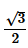times its initial speed. If the range of the projectileis P times the maximum height attained by it, P isequal to

Solution:
QUESTION: 33

The trajectory of a projectile in a vertical plane is y = ax -bx2, where a and b are constants andx and y are, respectively, horizontal and vertical distances of the projectile from the point of projection.The maximum height attained by the particle and the angle of projection from the horizontal area

Solution:
QUESTION: 34

A projectile is given an initial velocity of iˆ + 2 ˆj. The cartesian equation of its path is (g = 10 ms-2).

Solution:
QUESTION: 35

A particle is projected from the ground with an initial speed of v at an angle θ with horizontal. The average velocity of the particle between its point of projection and highest point of trajectory is

Solution:
QUESTION: 36

Two balls A and B are thrown with speeds u and u/2 ,respectively. Both the balls cover the same horizontal distance before returning to the plane of projection. Ifthe angle of projection of ball B is 15° with the horizontal, then the angle of projection of A is

Solution:
QUESTION: 37

A body of mass m is projected horizontally with avelocity v from the top of a tower of height h and itreaches the ground at a distance x from the foot of thetower. If a second body of mass 2 m is projectedhorizontally from the top of a tower of height 2 h, itreaches the ground at a distance 2x from the foot ofthe tower. The horizontal velocity of the second body is

Solution:
QUESTION: 38

A car is moving horizontally along a straight line with auniform velocity of 25 ms-1. A projectile is to be fired from this car in such a way that it will return to it after it has moved 100 m. The speed of the projection must be

Solution:
QUESTION: 39

The horizontal range and maximum height at tained by a projectile are R and H, respectively. If a constant horizontal acceleration a = g/4 is imparted to the projectile due to wind, then its horizontal range and maximum height will be

Solution:
QUESTION: 40

A particle is projected with a certain velocity at anangle α above the horizontal from the foot of aninclined plane of inclination 30°. If the article strikesthe plane normally, then α is equal to

Solution: